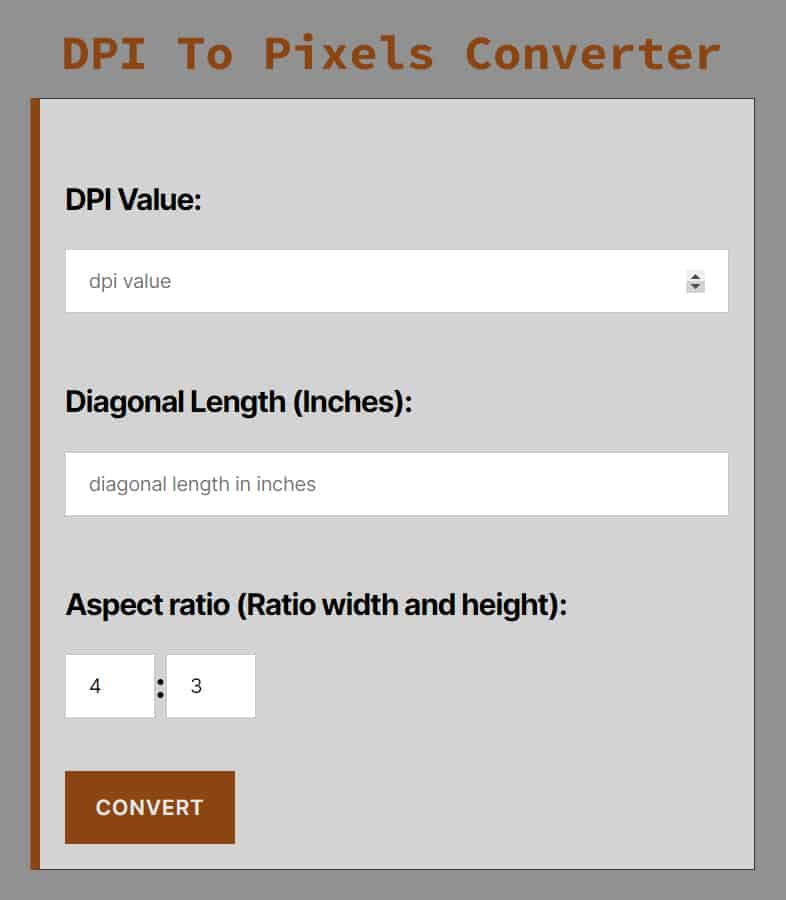# DPI To Pixels Converter

#### DPI To Pixels Converter

This is an online converter which you can use to convert dpi to pixels (px). Convert dots per inch (dpi) to px professionally for free.

##### Aspect ratio (Ratio width and height):

:

DPI is used to measure the resolution of an image both on screen and in print.

As the name suggests, DPI measures how many dots fit in a linear inch. Therefore, the higher in DPI, the more details can be displayed in an image.

#### How to use DPI to pixels converter

First, enter dpi value

Second, enter diagonal length in inches

Third, enter aspect ratio value

Finally, click on convert button#### How to calculate pixels from dpi

To convert dpi to pixels, there are two common methods, one of them the online converter we provide above, and the other is using dpi to pixels conversion formula.

#### DPI To Pixels Conversion Formula

As we mentioned above, to convert dpi to px you can use the converter or the formula.

To convert dpi to px you need three formulas:

First formula for calculating diagonal length in pixels

Diagonal length (pixels) = DPI * diagonal length (inches)

Second formula for calculating height in pixel

Height (pixels) = Diagonal length (pixels)/√(Aspect ratio²+1)

Third formula for calculating width in pixels

Width (pixels) = Diagonal length (pixels)/√((1/Aspect ratio²)+1)

Converting dpi to pixels can be done better using the online converter, because it is fast, accurate and easy to use.